Bokaro P ni Naritai, vol. 10

(Images used for review purposes only.)

I want to be a Vocaloid Producer, vol. 10, 1,500 yen, plus tax.
One of the interesting things about the Vocaloid concept is that the characters representing the voices, such as Miku Hatsune and Rana, are virtual robots. As robots, they’re supposed to have serial numbers, generally displayed on the cheek just below the left eye. This means that Rana specifically, in my case, can have a unique serial that shows up in whatever video or still image I create, marking that product as mine. In fact, I did follow the instructions in volume 1 to get my assigned number, which I wrote down, but I didn’t see anything telling me how to implement it in the model. A couple days ago, I got tired of seeing the black bar under her eye in my classwork, so I went back to the first magazine to try again. This time, I figured out what I should have done – download the .png file generated by the online number assignment page, rename it to “number.png”, and save it in the Rana directory, overwriting the existing black bar file. There was a new version of the model data announced in volume 7, available online, and the number.png file needs to be copied into the correct directory with each upgrade. But, I am happier with how Rana looks now.

New magazine features:
In the 4-panel comic, Rana visits a new class room – the Dance Room, where she exhausts herself in the first 30 minutes. Robo-panda tries bribing her into exercising longer, and the bribe is to be revealed in the next magazine. The classroom chapter has Rana learning about piano intervals and perfect chords, plus we’re introduced to a new accessory – the Brownie Sax. The interview section is with song-writer/animator and speed Pop master cosMo@暴走P (CosMo@BousouP). The magazine describes the highlights of the DVD-ROM tutorials, and goes into additional discussion of motion curves for the Jasmine and Rana animation cycles. The final page talks about the Zola Project software package and introduces the Pickup Artist, Kuroma.

New DVD Features:
Pickup Artist: Kuroma. Song: Boku da (It’s Me)
“It’s Me” is another plain sound file with no video or stills. The opening has a very strong retro-video game feel, with 8-bit style beeps and boops. The song settles down into more of a J-Pop format while Rana sings, but her voice has been tweaked to sound filtered or auto-tuned. As described by the magazine, this is an example of the “speed Pop” genre. It’s interesting, but all of the game sound effects are very distracting.

There’s one MMD accessory this time – the Dance Room. According to the magazine, the conceit is that there are 12 rooms heading off from the main warp room. The dance room is the third one, and is specialized to have motion capture cameras in the ceiling and walls, 8-speaker surround sound, and wide screen HD TV monitors for video playback to allow the Vocaloid to review his or dance moves. The accessory directory includes a new door skin with the number “03” on it, to replace the old skin in the Warp Room directory.(Page on using motion curves to give Jasmine a “bouncing ball” movement.)

Tutorials:
Vocaloid:
The past few tutorials demonstrated the use of the vocal style controls, including Velocity and Dynamics. In vol. 10, the remaining controls are described, and three of them – Gender, Clearness and Mouth Opening – are used to make Rana sound more boyish for the lyric “honki dashitenai” (I haven’t gotten serious yet”). The full set of controls are: Velocity, Dynamics, Breathiness, Brightness, Clearness, Opening, Gender, Portemento Timing and Pitch Bend. Most of them are self-explanatory. Then, as with the other tutorials, the remaining time is spent replaying the full demo song, titled “Vocaloid no Yuuwaku” (Vocaloid’s Temptation/Seduction). Because the finished songs are supplied with the DVD-ROM, it’s really not necessary to put them in the tutorial as well. It’s just to pad it out past the rather short 4 minutes and 30 seconds…

SSW:
SSW
sort of builds on what we learn in the Vocaloid tutorial, with the introduction of Distortion for the base guitar and Rana’s vocal track, and the use of the Maximizer on the final mixer output. Distortion acts kind of like a frequency filter, while the maximizer/cut-off is apparently a volume amplifier, clipper. Again, the tutorial ends with a replay of the finished song.MMD:
Vol. 10 builds on the up-down dance cycle, discussing motion curves in more detail and just suggesting what the student should do in applying all the concepts to the other three group members (Teacher Robo-panda, Teacher Jasmine, and the speaker box, Satchan). You can apply a motion curve to any keyframe, for x, y, z and rotation inbetweens. A straight line indicates linear movement from the previous key to the current key. An s-curve means you’ll start the movement slowly, speed up in the middle, and slow down at the end (or vice versa). Convex and concave curves can either give you a circular movement, or if mirror-flipped to be concave for one key and convex for the next, a bouncing ball effect (fast movement on the bounce, slow at the top of the arc).

The tutorial spends a lot of time showing the different motion curve approaches, but almost no time on the steps needed for importing Robo-panda, Jasmine, Satchan or the dance stage, into the project, or for positioning the new characters and setting key frames. The assumption is that anything not explained in the magazine has already been covered in the previous tutorials. The last few minutes of the tutorial consists of the finished music video created by the publishers, if you want to take their hints and make your own movie with camera movements and lighting effects. The tutorials have all been ending with “please experiment with the controls and upload your own works to niconico douga”.The demo song for the Vocaloid tutorial is an example of speed Pop. The music itself is a somewhat faster pop piece, but it’s the vocals sections, which consist of bursts of nearly unintelligible words packed into short spaces, that sets this genre apart from normal girl idol pop.

Complex Unsnarling

I want to explain a little more what was happening in the two videos – Snarl Dance and Complex Snarl – from the last post.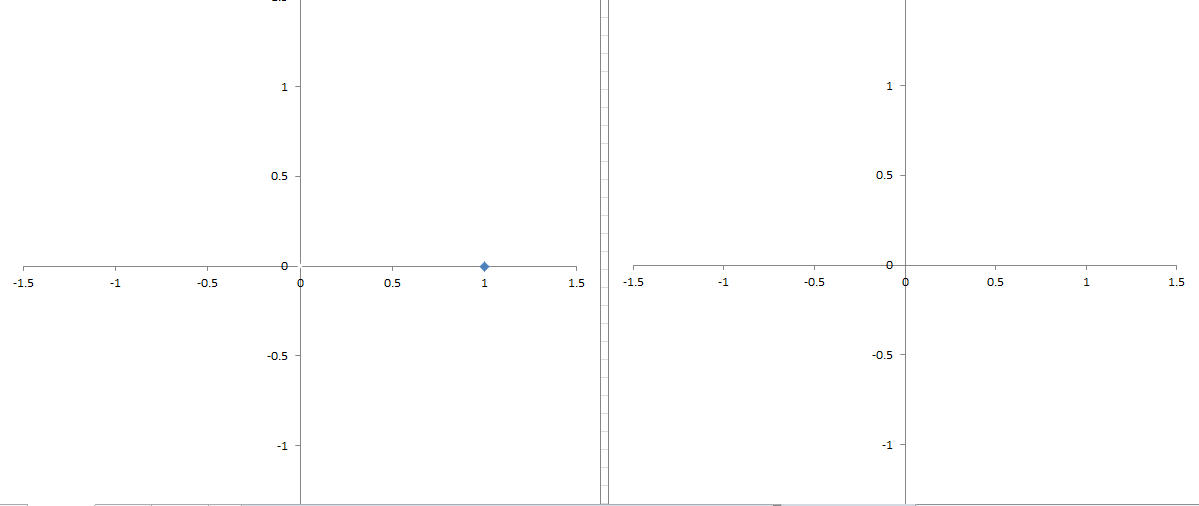(Graph 1: Left: y1=c^x1. Right: y2=c^2^x2. For x1=0, x2=-1.)

It’s a little hard to see, so I apologize. I spent several hours trying to get Excel to format the plots correctly because it couldn’t deal with 64K worth of data points, and I don’t really want to go through that again to make the lines thicker. If you want to get a better image, just click on the picture to get to the source file. Anyway, I specifically chose a+ib at (0.5+i*0.86) to create a spiral that decays very slowly. It’s pretty close to a circle, but after x1 reaches 65,536, y1 is (-1*10^-149 – i*7*10^-150), which is more or less 0.

We have two plots in Graph 1. The left plot is for y1=c^x1, where x1=0. By definition, this has a value of 1. The right plot is for y2=c^2^x2, which is the basic formula for the Mandelbrot fractal space. I won’t start plotting y2 until the first iteration of the left-hand formula.(Graph 2: Left, x1=1; right, x2=0)

In Graph 2, the left plot shows x1=1, and we’ve started on our death spiral. On the right, we have the data point we’d start with for the fractal, which is (0.5+i0.86). Note that here, both plots are using the same value, but y1=c^1 already has one calculation completed.

Excel wants to draw a curve between points, but doesn’t have enough data yet to get the curve correct. It’s starting to look like a circle, though (maybe). y1=c^2 and y2= c^2^1 = c^2. The only real difference between the two curves at this stage is that the one on the right didn’t start at 1+i0.(Graph 4: x1=4; x2=2)

Ok, what’s happening now is that we’re essentially sampling our curve on the left to get the data points for the curve on the right. When x2=2, c^2^x2 equals c^2^2, equals c^4. That is, we’ve taken 4 steps along our spiral on the left, and chosen to use that 4th value for the 2nd step on the “chaotic” line on the right. This line only looks chaotic because we’re losing the underlying logic of the spiral by taking exponentially larger sampling steps.

The sampling step size pattern is going to follow 2^x, for x from 1 to some upper bound. In other words, 2, 4, 8, 16, 32, 64, etc. 2^3 = 8, so our third data point on the right is actually the 8th point on the left. Unfortunately, for the starting value I’ve selected, this is very close to point one. For the rotation angle we have for this spiral, we’re essentially going to be bouncing between points 1 and 2 as the magnitude decreases, but with a small rotational offset.

Again, because the spiral takes 6 steps to complete one rotation, and our next sample is at point 16, we’re hitting almost the same two points, to give a “bouncing effect” to the curve on the right. If I’d picked some other value for c, the results on the right would be different (ref. Graph 8 below.)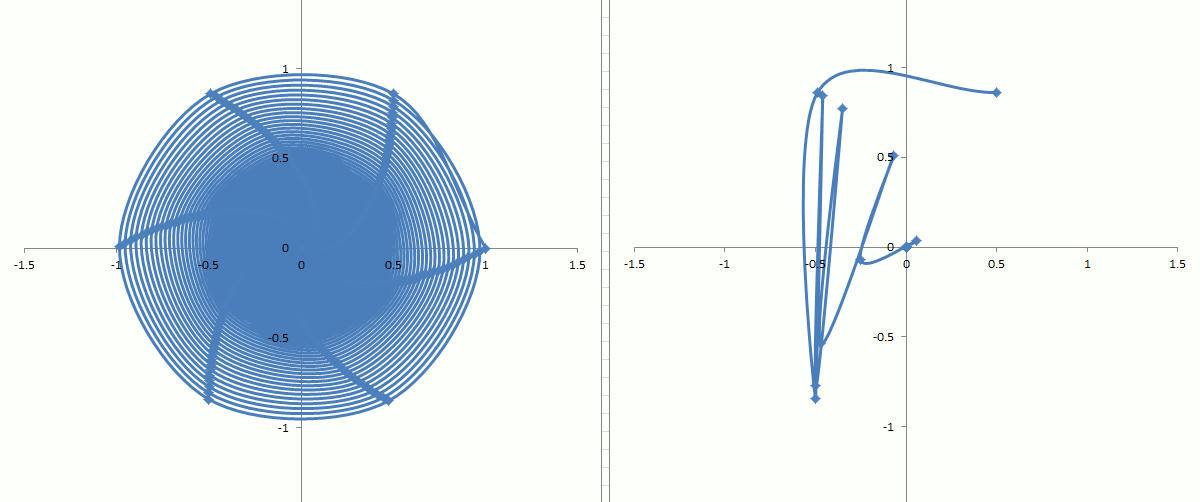(Graph 7: x1=65,536; x2=16)

I’ve kind of decided to jump forward a bit to where x2 is 16. This gives me my 16th sample, which is going to be data point 2^16=65,536 from the plot on the left. The death spiral has lived up to its name, and y1 has gone to 0, requiring that y2 does the same thing. Looking at both plots, it’s pretty obvious that the magnitude of y2 (sqrt(a^2 + b^2)) never exceeds 2. If we were drawing a fractal, we’d time out at x2=50 and color the point 0.5+i*0.86 black.(Graph 8: c=0.0954 + i*0.99542)

As promised above, Graph 8 shows what happens if you use a different starting value for c (in this case, a+ib = 0.0954 + i*0.99542). This point is very close to lying on a circle. y=c^x still decays to 0, but even after x1 reaches 65,536, y1 isn’t at 0 yet. The plot for y2=c^2^x2 is much more chaotic because this is the kind of image I wanted this time. On the other hand, the magnitude of y again never exceeds 2, so in a fractal, this point would still be colored black. If you’re drawing fractals, then all the really interesting action takes place when the starting value of c lies on a spiral that just barely tends to infinity. The closer that spiral is to a circle, while remaining unbounded, the prettier the resulting fractal images are.

Bokaro P ni Naritai, vol. 9

(Images used for review purposes only.)

I want to be a Vocaloid Producer, vol. 9, 1,500 yen, plus tax.
New magazine features:

New DVD Features:
Pop-Up Song: Layla, by Mushi-P
The video is actually just a still from a pop-up picture book. The song itself is a bouncy little J-Pop piece which is dominated by a xylophone back-up. Not bad, but I’m not sure I’d want to listen to it more than once or twice a week. Certainly NOT in heavy rotation play on the radio…(Rana and the rest of the cast talking about idol dance moves.)

Tutorials:
Vocaloid:
In vol. 7 and 8, nuance and accent were applied to Rana’s vocals on a note by note basis. In vol. 9, we use Divide Selected Part to break Rana’s vocals into two sections, and Settings->Singing Style to apply very short syllable emphasis to the entire first half of the song in one single action. Afterward, Part->Join Selected Parts stitches the two halves back together again. This is followed by instructions to change DYN on Rana’s vocals to soften her voice when the music changes to a gentler tempo.

SSW:
The main focus in this tutorial is the use of copy-paste to duplicate Rana’s vocals to get a repetition of one word a couple beats apart, and then using the phrase editor to insert drum and tambourine phrases into the demo song. Largely, the demo is a “wall of sound” where little bits and pieces, like a drum riff here, or an explosion sound effect there, are stuffed into blank sections of the music to fill them out much like you would with “bucket fill” in Photoshop. Again, it’s the Japanese cookie-cutter approach to J-Pop composition, where the music often clashes with the vocals due to “dueling” genres.(Still from Rana’s dance cycle.)

MMD:
And, we also get a little bit of theory on J-Pop Idol dancing. The idea is that in a rhythmic motion, such as bopping up and down, the dancer can either be standing up on the beat and crouching in between (“up rhythm”) or crouching on the beat (“down rhythm”). Most idol dances are down rhythm patterns. The rest of the tutorial describes how to set the keyframes for a down rhythm dance cycle, and the use of the motion curve window to make Rana’s actions less linear (slowing down at the top and bottom ends of the cycle). The steps themselves are pretty straight-forward, since the “up” and “down” poses are supplied with the DVD, and it’s just a matter of applying them at the given key frames. The last part of the video has you importing the crab headgear and anchoring it so it follows Rana’s movements as she dances.

Again, I can output the video as an .AVI, but not as a bitmap. This indicates that there’s something in the camera or lighting setups that hasn’t been detailed in the tutorials yet. So, to make the above still, I saved the video to an .AVI, then used print screen to copy-paste one frame into Gimp for editing. MMD does allow for adding background pictures and .AVIs, so I’d imported a random image I got from a Japanese beer magazine (image by Jesse Kercheval) to make Rana stand out better.

The above joke from the 4-panel comic revolves around the word for money. On New Year’s Day, it’s traditional in Japan for older family members to give small envelopes of money (called Otoshidama) to the younger members. The word for money is “kane”, or often “o-kane” with the addition of the honorific “o-” prefix. Rana is trying to wheedle Robo-panda into giving her “O-kane”, and instead receives the “kane” gift, since “kane” is also the word for “crab”.

Complex Snarl Dancing

In the last post, I talked about complex math giving me pictures that I like playing with. I decided to make two animations in Excel that I could put on youtube. I made the music using the Hackme Rock-it synth kit.

The first one is Complex Snarl.

If you have the formula y = c^x, you’re going to get a spiral that either goes to 0, or to infinity, depending on your value of c. This is the basis of the Mandelbrot fractal, which uses cn+1 = cn^2, and assigns a color to the starting value of c0 given the value of n where the magnitude of cn is greater than 2. If n reaches 50, then stop and color that point black. The thing is, the Mandelbrot form is the same as y = c^2^x, or y = c^x for x = 1, 2, 4, 8, 16, 32, etc. That is, you’re skipping points along the curve that you’d get if you plotted y = c^x. So, instead of getting a nice clean spiral (y = c^x), you’re getting something more chaotic looking (y = c^2^x) only because you’re sampling points at exponential intervals along that spiral.

So, what happens if you plot y = c^x? Answer, it depends on what you pick for c.

If c = 2 + i*0, then you get y = 2^x, which is an exponential curve racing to infinity as x gets bigger.
If c = 0.5 + i*0, then you get y = 0.5^x, and a short little arc that goes to 0.
If c = 0.43 + i*0.9, it’s a spiral that goes to 0 as x goes to infinity.

As “a” moves from 0.43 to 0.43588989435407, with b fixed at 0.9, the spiral unwraps, taking longer for y to approach 0 as x gets bigger. When a=0.43588989435406, y is right on the brink of turning into a circle. At a=0.43588989435407, y suddenly spirals the other way, heading off to infinity. What I did was to animate the plots for y = c^2^x, with x = 0 to 340, as “a” approaches, then hits this discontinuity.

Snarl Dance is y = c^2^x, where I’m changing “b” from 0 to 0.9, while keeping “a” fixed.

Bokaro P ni Naritai, vol. 8

(Images used for review purposes only.)

I want to be a Vocaloid Producer, vol. 8, 1,500 yen, plus tax.
One of the reasons I didn’t write up this review over 2 weeks ago is that my copy of the magazine for issue 7 didn’t include the serial numbers for renewing each of the software packages. This is a kind of nasty trick on the part of the publishers, making us buy the odd-numbered issues in order to extend the licenses for another month each time. I think the next serial number set should have been included with the 7th magazine, but all I got was a notice telling me to download the latest version of the Rana model files for Miku Miku Dance (and which I misunderstood to mean that the MMD app itself was being upgraded).

New magazine features:
4-panel comic telling us that Rana is having trouble reading sheet music, and the Crea school lesson on what “major scales” and “minor scales” are. Interview with Fuwari P, and a mention of some of his current songs. Plus, one page on how to open the source files for the Pick-Up Artist song, “Nigiiro Monster” (Rainbow-Colored Monster) in Vocaloid.

New DVD Features:
Pick-up Artist Song: Rainbow-Colored Monster, by 10 Day P-san.
With previous DVDs, clicking on the button for “Pick-Up Artist” launches Media Player to play a music video of some sort. This time, it opens Windows Explorer to reveal the Vocaloid source file for Rainbow-Colored Monster, which was the main demo song for volume 1. There’s also a .wav file for the finished exported file, plus a karaoke version. According to the magazine, the idea is to mess with the music source to see how the various tricks work.(Using MMD to pose Rana for a still photo.)

The magazine also introduces a PDF file included on the DVD – “Sakkyoku Dekiru” (I Can Compose Music). This is a 16-page instruction manual on how to use Vocaloid to create and orchestrate lyrics for a song from scratch. It’s in Japanese, of course, but there’s a lot of screen caps, and those are self-explanatory. The document does get into music theory a bit, correlating the sheet music layout with the keys on a piano.

Tutorials:
SSW:
There’s more than one way to get to certain sound controls in Singer-Songer Writer, when you want to modify the way a specific instrument plays during the song. This time, we use the strip editor to change Expression, or the timbre, of the MIDI strings track by drawing the magnitude waveform of the effect using straight lines, freehand draw, sinewaves or even “random” height (similar in approach to what’s been demonstrated in previous issues). The second half of the tutorial talks about Automation, but this seems to be just a different window within the instrument tracks display for drawing the waveforms for Volume, Panpot and Compression for the audio tracks (the example is to change the volume of Rana’s voice so it’s not drowned out by the drums or guitar over the course of the song).

Vocaloid:
This is a fairly short tutorial, probably because most of the work was put into making the Sakkyoku Dekiru PDF. Instructions involve using Bend to make Rana’s voice change pitch through part of a syllable, and cutting one bar of lyrics to paste them into a second track to deliberately emphasize a break in syllable pronunciation. The example uses the phoneme “fun”, which initially comes out sounding like “funa”. Cutting “fu”, putting it into track two and then changing DYN to zero out the pronunciation of the leading part of the syllable fixes this problem.(Still frame from Miku Miku Dance, using the Christmas set accessory from the DVD-ROM. I finally got still capturing to work!)

MMD:
While MMD is positioned as an animation package for making music videos, it is equally suited for generating 3D stills. The video is a bit harder to follow, largely because it skips over topics covered in earlier issues and, to be honest, the editing of the video isn’t very good at times. One case in point is when the captions block the menu pulldowns when we’re being shown how to change the screen size for saving a scene to a .png file. Another complaint is that the winter scene for the demo is pre-built, but the source files for the models are in directories that don’t match the user’s computer. There’s no guidance on how to find those models with the file browser. It took me a while to get the set and actors to show up right. I got it eventually, though. This time, saving a frame capture to a bitmap file DID WORK, but it’s apparently related to something about the lighting or camera position defined in the demo stage model and I have no idea what that something is. In the demo, Rana is positioned in the standard Japanese “cute girl pose”. I didn’t want to spend that much time on this project, so I kind of settled for a plain hack with a couple of stock hand poses from the supplied DVD-ROM (there are about 20 files on the DVD with poses saved for both hands, including fists, peace signs, and having the hand held wide open). The tutorial ends by telling the users to make their own artwork and videos with Rana and then upload them to Niconico Douga (the Japanese version of Youtube).

It’s amazing just how powerful and advanced all three software packages are. At some point, I’m going to have to knuckle down to try really mastering some of it.

Fractaling

While I’m working on my next approach to the Riemann Zeta (and if anyone knows the answer already, please let me know), I’d like to write a little bit more about complex numbers.

If you look at real numbers, they behave very predictably when you square them, which is essentially the same as drawing a square with side “x”, and then looking at “y” as the area of that square. With one specific exception, real numbers are going to fall into two categories: 1) The squares are going to get really big fast; or, 2) they’re going to get small really fast. The exception is x=1, where x^2 doesn’t change. This may not be obvious from the graph, but I’ll come back to this point.

If we take the next step and cube the number (x*x*x = x^3), which gives us the volume of a cube with side “x”, we get an interesting effect – when x is negative, the volume is negative. Or rather, the result of taking x to the power of 3 is negative for negative x. Regardless, y gets bigger, or smaller, a lot faster than when we just squared x.

Just for amusement value, I’ll include the graph for x^4.

And x^5. The greater the exponent, the more the curve flattens towards 0 between x= -0.5 and 0.5, and the more the sides straighten out for x > |+/- 1.5|. The corners right around +/- 1 will remain rounded, though.

This leads into an obvious question – what happens if we use non-integer steps for the power of x? That is, what if we did something like y = a^x, where x could go from 1.672 to 4.898? The answer is, if “a” is 1, nothing; y is a flat line. If “a” is greater than 2, y gets bigger nonlinearly and if it’s between 0 and 1, it gets smaller nonlinearly. If “a” is negative, Excel will give a “not a number” error for trying to take a negative root.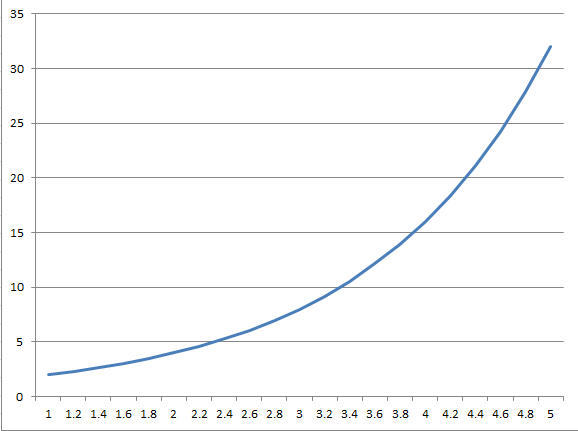(Graph 5: y = a^x, for a > 1 (a=2 in this case))(Graph 6: y = a^x, for a < 1 (a=0.5 in this case))

The following graph shows this point a bit better. y = a^x, where x runs from 1 to 5, and the lines are for differing values of “a” (from 0.9 to 1.1) As “a” approaches 1, the line for y flattens out, while the farther away it gets from 1, the greater the effect x has as an exponent, causing y to grow or shrink exponentially.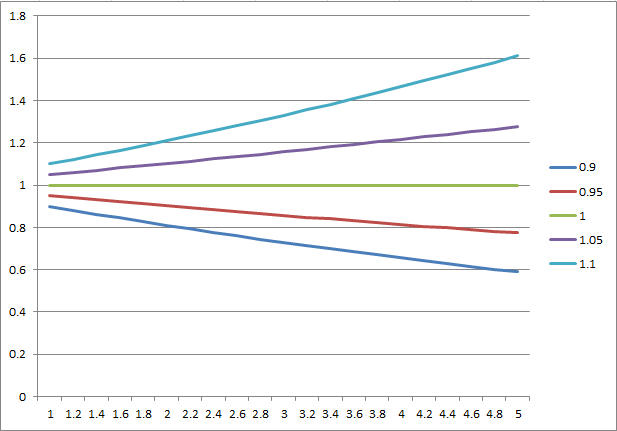(Graph 7: y = a^x, for differing values of “a” as x ranges from 1 to 5.)

Not really earth-shaking, but worth getting out of the way as quickly as possible. What this means is that, generally, exponents are continuous. A fractional exponent between 2 and 3 (such as 2.762) will fall on the curved line between 2^2 and 2^3 (2^2 < 2^2.762 < 2^3.) Sweeping x from 2.762 to 2.772 just causes y to smoothly flow along this line.

Now, what happens when we square a complex number? The above graphs could be said to be a special case for complex numbers “a + ib” where b = 0. So, let’s make “b” non-zero.

s^2 = (a +ib)^2 = a^2 + 2iab – b^2 = (a^2 – b^2) + i*(2ab)

We’re changing the magnitude of the point, while rotating it a certain amount around the origin. This leads to weird results. In the following chart, I have 5 lines. The bottom line is for “a” from 1 to 8, with “b”=0. For the second line, “b” = 1, and then incrementing “b” for the other lines. The graph itself is the result of (a +ib)^2. Increasing “b” causes the point (a+ib)^2 to rotate further, and the amount of rotation is nonlinear with respect to “a”.

Where the weirdness of the complex plane comes in is when we repeat the exercise from the real axis above – taking s^x powers for incrementally increasing x.(Graph 9: (2 + i1.5) ^ x, for x = 1, 2, 3, 4, 5.)

In this graph, s = 2 + i1.5. The first point is for x = 1. The second point is for (2 + i1.5) * (2 + i1.5) (that is, x = 2). The other points are for x = 3, 4 and 5. What should be obvious is that for larger values of x, y rotates in a spiral, and the magnitude for y is going towards infinity. Yes, this is kind of like what happened for real numbers, but there’s a “spin” involved, and the real portion of x (“a”), swings both positive and negative.

You may be familiar with the butterfly effect, which states that a butterfly flapping its wings in China can cause a hurricane to blow in North America. The original butterfly effect was discovered by Edward Lorenz in 1961 when he was trying to run a weather forecasting simulation on a mainframe computer. According to one story, he didn’t have a way of automatically recording the numbers he was printing out, so at night he’d shut the simulation down, and in the morning he’d start it up again by typing in the values he wanted to simulate from. The problem was that his program had a little bit more precision than the printout displayed, and after a few minutes, the simulator would be generating dramatically different results than what he expected, for what he thought was the exact same starting values.

What was happening in the simulation was that Lorenz kept repeating the same operations over and over again, and very small differences would be compounded over time. And, they were complex numbers, which provide a kind of feedback. In other words, very small variations in the starting positions of equations using complex numbers can add up if you repeat an operation often enough.

This leads me to Mandelbrot. Benoit Mandelbrot pretty much created fractal science single-handedly. The classic Mandelbrot set uses a formula similar to:

C1 = C0 * C0

What we’re doing is taking a starting complex number and squaring it. The result then becomes the next value that we square again. With real numbers, starting from 2, the sequences would be: 2, 4, 16, 256, 65,536, etc. It gets big fast. What we’d want is to locate the value of “a” that is bounded. Or, what is the boundary number where y starts going to infinity? The answer is 1. If x is less than 1, y will go to 0. If x is 1, y will remain 1. If x is greater than 1, y will go to infinity. 2 is greater than 1 (for most values of 2), so yes, r1 = r0 * r0 is not bounded when we start with r0=2.

Notice that r1 = r0^2 has an interesting property. If we look at r0=2, then 2, 4, 16, 256, 65,536, etc., can be rewritten as 2^1, 2^2, 2^4, 2^8, 2^16…

Or, 2^2^0, 2^2^1, 2^2^2, 2^2^3…, or y = 2^2^x. So we could generalize the original fractal formula as y = r0^2^x, where x is an integer.

There was a hypothesis that stated that if the magnitude of complex number c0 >= 2, then c0 * c0 would go to infinity. So, if you drew a circle of radius 2 around the origin, any complex point inside that circle, when squared, would stay within, or close to, that radius. However, as soon as the point escaped the circle, squaring it again would cause the result to go to infinity very quickly.

What Mandlebrot did was to color code the points within the complex plane based on how many times that point could be squared before the resulting magnitude exceeded 2. Because many of the points never escape the circle, there’s a cutoff – repeat the process up to 50 times and if it doesn’t escape, color that point black and go to the next point. When you draw fractals on a computer screen, you’re essentially drawing the screen as a complex plane as “a + ib”, and each pixel is one increment of “a” or “b” within the complex space you’ve chosen (such as, from (-2,-2i) to (2, 2i) for a screen 1400 pixels by 1000 pixels. “a” has a step increment of (2 – (-2)) /1400 = 0.0029. “b” increments 4/1000 = 0.004).

Again, the formula is:

C1 = C0 * C0,
where each new value of C1 becomes the next value of C0, until the magnitude of C1 > 2, or you’ve repeated the process 50 times. Color each point on the complex plane based on how many times you repeated the formula before stopping.

In Graph 9, (a + ib) = (2 + i1.5), the magnitude is already 2.5, and I’d have stopped at “n” steps = 0. Instead, let’s try (0.9 + i0.435).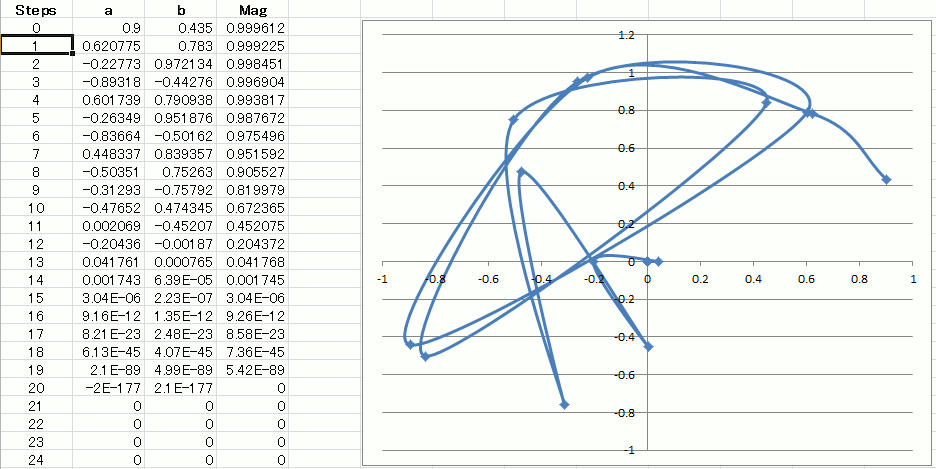(Graph 10: Plot of Cn+1 = Cn^2)

This is much more cool. Every time we square Cn (starting with Cn0 = 0.9 + i0.435 and then making Cn the old Cn+1) it runs off in a different direction before eventually decaying to 0 + i0. The really cool parts are when Cn doesn’t decay, and just seems to stay in a holding pattern.

Now, compare Cn+1 = Cn*Cn to y = Cn0^2^x.

It’s really the same thing. We’re just stopping after each increment of x to see if the magnitude of y is greater than 2. When we color code that point for Cn0, “x” is the same as “n”, the number of times we repeated the squaring operation. If we stop at 50 and give up for that point, it’s like we have squared Cn0 2^50 times. That is, we’ve multiplied Cn0 by itself trillions and trillions of times, and either it’s gone to 0 already, or it just refuses to grow up. Yet, if we move to the next pixel on the screen so that Cn0 = a + 0.0029 + ib, the results are going to be completely different. This is the butterfly effect – small differences in starting value get compounded over time.

The reason the curve for Cn+1 = Cn^2 looks so chaotic is that we’re skipping points in the middle. As shown in graphs 8 and 9, the imaginary part of a complex number acts as a discrete phase shift when we multiply complex numbers together. Multiplying Cn0 by itself creates an expanding spiral. Cn0^x (where x is a real integer, 1, 2, 3, 4, 5, 6, 7, 8…) is a spiral of disconnected dots. Cn+1 = Cn^2 skips a LOT of the points inbetween, because x is effectively increasing as 2, 4, 16, 256, 65,536… The spiraling effect is made completely invisible.

Why mention any of this at all? Well, the Riemann Zeta function performs a kind of similar operation on each value of “n” within the summation 1/(n^(a+ib)). True, they’re not really the same operations, but the Zeta function is hard to visualize while the Mandlebrot fractal is easier. Besides, I really like fractals and I like talking about them. It gives me an excuse to make new art projects, so there.

Riemann prose, part 8

Some time ago, I was talking (writing) about finding the area of a backyard, and I said (wrote) something about taking unit steps, and adding up all of the lengths of the unit steps.

In my example, I used a yard 40’x100′. So,
40*1 + 40*1 + 40*1 … 100 times.

In taking narrower and narrower steps, we get an approximation closer to the actual area underneath a curve. If the curve is y = 5, for x from 0 to 5 (5 steps of unit 1),

area under the curve = 5+5+5+5+5 = 25

Generalized, the area under y = x, for n from 0 to x is

x * x = x^2

If the curve is: y = 3x, and we go from 0 to 4:

area(y) = 0+3+6+9+12 = 30 (because the steps are so big, we introduce an error of 4 * 3/2, or 6.)

Generalized, the area under a triangle y = ax, for n from 0 to b;

1/2 a*b^2

This is the start of integral calculus. If we take an infinite number of infinitely thin slices, we will get the precise value of the area under whatever curve we pick (ignoring the exceptions).

And, this where sigma comes in. Sigma represents the addition of a string of values from some lower limit up to the upper limit.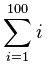The above equation just tells us to add the positive integers from 1 to 100 (5050).

So, looking at the Riemann Zeta function one more time,We’re going to sum a formula from 1 to infinity, where the formula uses a complex value, “s”, and “s” is in the form of “a+ib” in the complex plane. For each value of “n”, we will raise “n” to the complex exponent and take the reciprocal (divide 1 by that number). “n^s” is the same as:

n^a * (cos(ln(n^b)) + i*sin(ln(n^b)))

Yeah, so what? How do we draw this?

Basically, we’re going to look at the entire complex plane as one big sheet, then make it three dimensional by adding a z-axis, where z is the total of all the sums for each point “a+ib”.

What this is going to do is to give us a ripply, wavy sheet that sometimes goes to negative z, sometimes holds at a fixed value (converges) and sometimes goes to positive infinity on the z-axis.

Why? Why do we care?

What got me started is the Riemann Hypothesis, which says that the Riemann Zeta function equals 0 when, and only when, the real part of s = 1/2 (a is the square root).

Actually, there are two sets of places where the zeta function equals 0, the “trivial zeros” and the “non-trivial zeros”. The trivial zeros are at s = -2, -4, -6, etc. (“a” is a negative even integer, and “b” is 0).

The non-trivial zeros are all in the “critical strip” where “a” is close to 1/2, but no one’s been able to prove mathematically that they’re all actually on the “critical line” of a=1/2. The first trillion non-trivial zeros have been calculated with a computer, and they’re all on the critical line, but calculating them is not the same as proving the hypothesis.

Now, if only it were that easy.

If we add up a series of numbers and the total goes to infinity, then the series is said to “not converge”. Conversely, if the total keeps getting closer to some value without exceeding that, the series is said to converge at that value.

x = 1 + 2 + 3… etc. goes to infinity.
x = 1/1 + 1/2 + 1/3 + 1/4… still goes to infinity, only slower
x = 1/1 + 1/4 + 1/9 + 1/16 * 1/25… converges to about 1.64

The faster the farther values get smaller, the more likely the total is to be convergent.

The problem is that the Riemann Zeta function is not convergent when “a” is < 1.

Let me repeat that – the Zeta function as given above goes to infinity when the real part of s is less than 1. (And, 1/2 is less than 1, the last time I checked.)

This is why mathematicians will use other forms of the equation to find the Riemann Zeros, specifically the analytic continuation. Unfortunately, I haven’t wrapped my head around that yet.

The formula I came up with:

f(x) = sum(1/(n^a * cos(ln(n^b) + n^a*i*sin(ln(n^b))))

doesn’t work. (sob) But it does make pretty pictures.

For x = (0.5 + i* 14.13):

At the first non-trivial zero, this version of the Riemann Zeta just keeps getting bigger as you continue summing up the n^s parts. It doesn’t converge to (0, 0).

(Graph of the zeta function, with a = 1/2, and i*b from 1.8 to 15. Nope, zeros here.)

I kind of thought I was doing it wrong, since we usually see formulas using sines and cosines as cos(2 * PI * x). So, I changed the spreadsheet to use 2*PI. The sum converges, but not to (0, 0).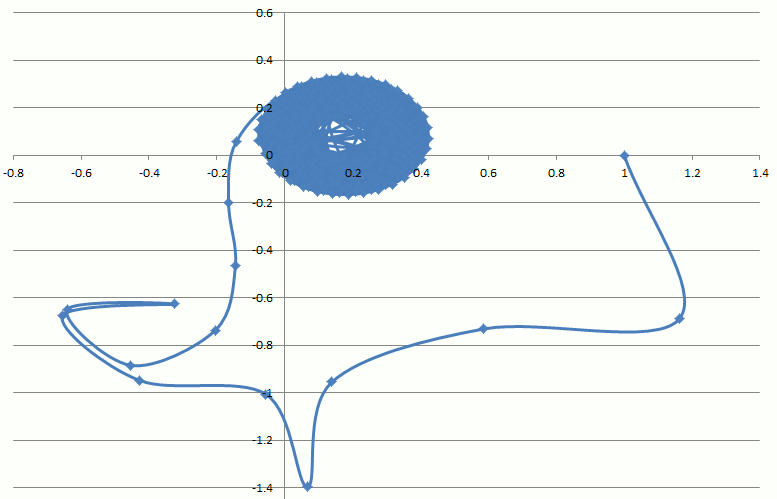The real and imaginary parts just don’t both go to 0 simultaneously, which is a bad thing for the home team.